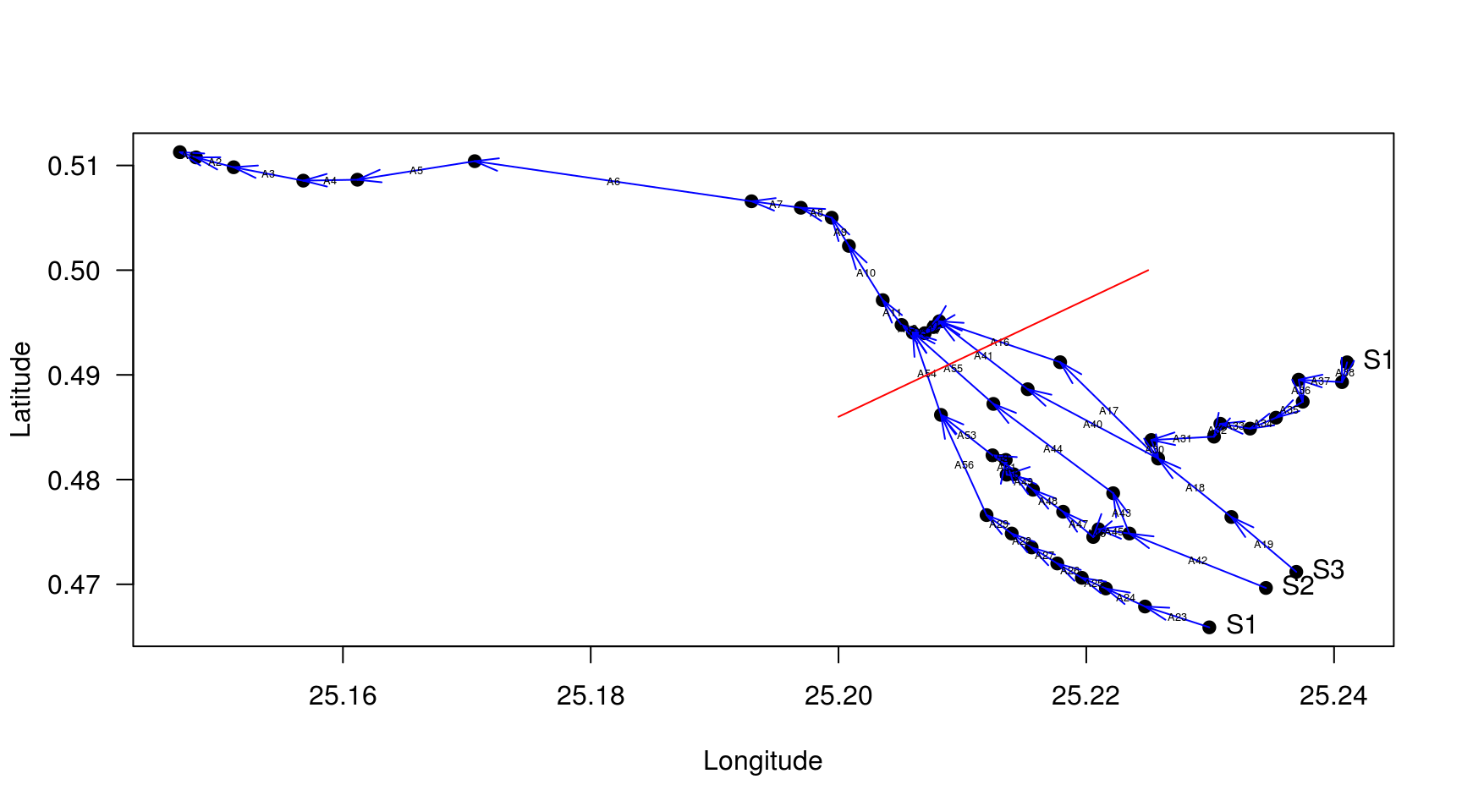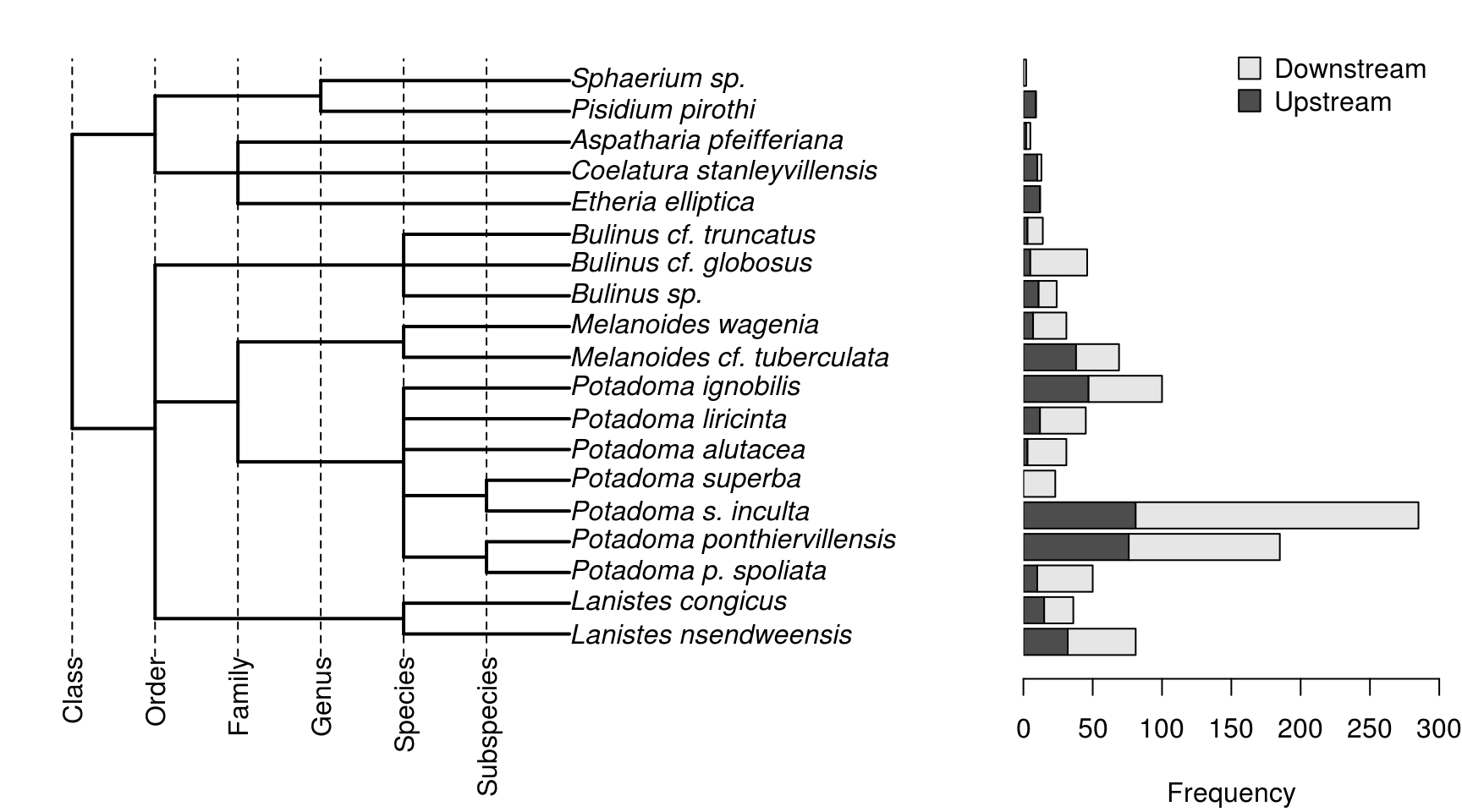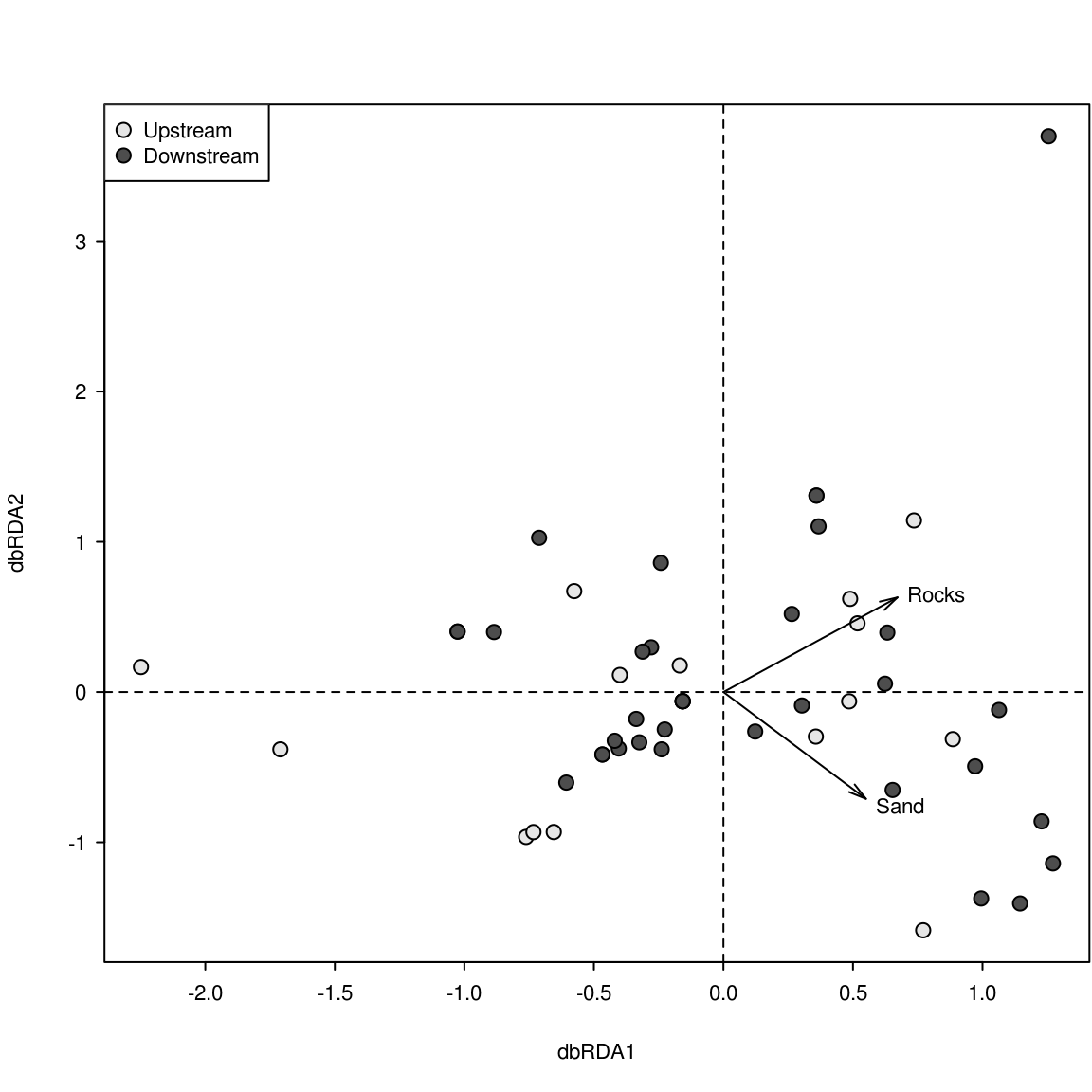Oscar Wembo Ndeo1,2,3\$; Torsten Hauffe2; Diana Delicado2; Alidor Kankonda Busanga1 & Christian Albrecht2,3

1Department of Hydrobiology and Aquaculture, University of Kisangani, BP 2012, Kisangani, D. R. Congo.

2Department of Animal Ecology and Systematics, Justus Liebig University Giessen, Heinrich-Buff-Ring 26-32 (IFZ), D-35392 Giessen, Germany.

3Department of Biology, Faculty of Science Mbarara University of Science and Technology, P. O. Box 1410, Mbarara, Uganda.

\$Corresponding author: wemboscar@googlemail.com

This html document is reproducing all steps of the statistical analysis performed in the manuscript.

Load necessary R packages:

``````library(vegan)
library(ape)
library(raster)
library(rgdal)
library(gdistance)
library(AEM)``````

Load the raw data. These consist of

• the supplementary Table 1 with all species counts, environmental features, and the connectivity matrix linking the sampling localities
• the GIS shapefile for calculating the watercourse distance among sampling localities
``````Comm <- read.table("SupplementaryTab1.csv", header = TRUE, sep ="\t", row.names = 1)
# Create shapefile of the study area:
Congo <- matrix(c(25.14571, 0.5126965, 25.15030, 0.5110112, 25.15383, 0.5099388,
25.15881, 0.5093260, 25.16325, 0.5095558, 25.17083, 0.5112410,
25.17666, 0.5101686, 25.18271, 0.5090195, 25.18761, 0.5071810,
25.19044, 0.5066448, 25.19458, 0.5072576, 25.19780, 0.5069512,
25.20079, 0.5051127, 25.20232, 0.5019720, 25.20354, 0.4987546,
25.20477, 0.4967629, 25.20599, 0.4955373, 25.20737, 0.4959969,
25.20898, 0.4957671, 25.21051, 0.4947712, 25.21373, 0.4942350,
25.21672, 0.4932391, 25.21886, 0.4918603, 25.22024, 0.4890259,
25.22055, 0.4865746, 25.22285, 0.4841233, 25.22430, 0.4845063,
25.22606, 0.4852723, 25.22921, 0.4848893, 25.23150, 0.4864214,
25.23357, 0.4871108, 25.23518, 0.4881833, 25.23595, 0.4899452,
25.23786, 0.4909410, 25.24001, 0.4920901, 25.24207, 0.4923199,
25.24253, 0.4910942, 25.24276, 0.4886429, 25.24031, 0.4878769,
25.23794, 0.4862682, 25.23464, 0.4843531, 25.23081, 0.4832806,
25.22798, 0.4819784, 25.22675, 0.4811357, 25.22966, 0.4787610,
25.23250, 0.4773055, 25.23472, 0.4753138, 25.23740, 0.4733987,
25.23870, 0.4717901, 25.23832, 0.4691089, 25.23204, 0.4637466,
25.22469, 0.4667342, 25.21848, 0.4699516, 25.21417, 0.4728421,
25.21074, 0.4763097, 25.20703, 0.4814621, 25.20546, 0.4857320,
25.20091, 0.4956499, 25.19802, 0.4996765, 25.19251, 0.5025082,
25.18018, 0.5044233, 25.16670, 0.5022018, 25.15605, 0.5003633,
25.14548, 0.5024316, 25.14126, 0.5053425, 25.14149, 0.5096324,
25.14571, 0.5126965), ncol = 2, byrow = TRUE)
Hole <- matrix(c(25.21111, 0.4893689, 25.20879, 0.4884882, 25.20959, 0.4859261,
25.21279, 0.4831238, 25.21439, 0.4820829, 25.21528, 0.4800012,
25.21688, 0.4789604, 25.21840, 0.4778394, 25.21984, 0.4762381,
25.22064, 0.4761581, 25.22264, 0.4756777, 25.22320, 0.4767185,
25.22104, 0.4789604, 25.22048, 0.4805617, 25.21888, 0.4824833,
25.21463, 0.4850454, 25.21207, 0.4864065, 25.21199, 0.4885683,
25.21111, 0.4893689), ncol = 2, byrow = TRUE)
Hole <- Polygon(Hole, hole = TRUE)
Congo <- Polygon(Congo, hole = FALSE)
Congo4Distance <- Polygons( list(Congo, Hole), ID = "a")
Congo4Distance <- SpatialPolygons(list(Congo4Distance),
proj4string=CRS("+proj=longlat +datum=WGS84
+no_defs +ellps=WGS84 +towgs84=0,0,0"))
Connectivity <- Comm[,34:89]

sum(Comm[,1:19])``````
``##  1061``
``````CommOscar <- Comm[,1:19] # Take just the species
CommOscar <- ifelse(CommOscar > 0, 1, 0) # Convert abundancies to presence absence``````

### Five different set of predictors of mollusk community composition

#### The first two sets (‘Environment’ and ‘Barrier’) are already included in supplementary Table 1.

The following chunks create the spatial Eigenvector maps of the last three sets of predictors.

All of them need the watercourse distances among sampling localities. First, the GIS shapefile of the sampling area and the geographic coordinates of the sampling localities are transformed to an equal distance projection. This avoids distortions because distance of 1? in longitute does not equal 1? in latitude. Although at the Equator, this should not matter.

``````# Equal distance transformation
CongoAeqd <- spTransform(Congo4Distance, CRSobj = CRS(
"+proj=aeqd +lat_0=0.48 +lon_0=25.19
+ellps=WGS84 +datum=WGS84 +units=m +no_defs+towgs84=0,0,0"))
CongoRas <- raster(xmn=bbox(CongoAeqd)[1,1], xmx=bbox(CongoAeqd)[1,2],
ymn=bbox(CongoAeqd)[2,1],ymx=bbox(CongoAeqd)[2,2],
crs=CongoAeqd@proj4string)
res(CongoRas) <- 10 # Grain size
CongoRas <- rasterize(CongoAeqd, CongoRas)
CongoTrans <- transition(CongoRas, mean, 16) # Lasts a bit...
# Equal distance transformation of sampling localities
CoordGcs <- SpatialPoints(Comm[,c("Longitude","Latitude")], proj4string = CRS(
"+proj=longlat +datum=WGS84 +no_defs +ellps=WGS84 +towgs84=0,0,0"))
CoordAeqd <- spTransform(CoordGcs, CRSobj = CRS(
"+proj=aeqd +lat_0=0.48 +lon_0=25.19
+ellps=WGS84 +datum=WGS84 +units=m +no_defs+towgs84=0,0,0"))
# Watercourse distances
CostDist <- costDistance(CongoTrans, CoordAeqd)
CostDist <- as.matrix(CostDist)``````

#### Third set of predictors (‘Non-directional spatial’)

The distance-based Moran’s eigenvector maps are computed in vegan.

``````# dbMEM
Mem <- pcnm(CostDist)
MemMor <- Mem\$values
MemVectors <- as.data.frame(Mem\$vectors)``````

#### Fourth set of predictors (‘Downstream-directed spatial’)

This chunk of code follows the instructions of Borcard et al., 2011: Numerical ecology with R, page 280 for constructing Asymetric Eigenvector Maps (AEMs). The manually edited connectivity matrix was already loaded at the very beginning. The links between the imaginary sites connecting to S1-S3 and S13 for imposing directionality are removed first. This workflow equals the behavior of the `aem` function with the argument `rm.link0 = TRUE`, removing all zero links.

``````# Equals the behavior of aem(..., rm.link0=TRUE):
Connectivity <- Connectivity[,-which(colnames(Connectivity) %in% c("A20", "A21", "A22", "A39"))]
# Edges according to row-order in the connectivity table
Edges <- rbind(c(29,51), c(42,13), c(51,13), c(50,51),
c(49,50), c(48,49), c(47,48), c(46,47),
c(45,46), c(44,45), c(43,44), c(40,43),
c(41,42), c(40,41), c(21,40), c(39,16),
c(18,39), c(38,37), c(37,36), c(36,35),
c(35,34), c(34,33), c(33,32), c(32,31),
c(31,30), c(30,18), c(28,29), c(27,28),
c(26,27), c(25,26), c(24,25), c(23,24),
c(22,23), c(20,19), c(19,18), c(18,17),
c(17,16), c(16,15), c(15,14), c(14,13),
c(13,12), c(12,11), c(11,10), c(10,9),
c(9,8), c(8,7), c(7,6), c(6,5), c(5,4),
c(4,3), c(3,2), c(2,1))
Edges <- Edges[nrow(Edges):1,] # Reverse because connectivity matrix is the other way around.
# Weights are the watercourse distance and need to have the same length than ncol(Connectivity)
EdgeDistances <- apply(Edges, 1, function(x) CostDist[x,x]) # Length of the edge
Weights <- 1 - (EdgeDistances/max(EdgeDistances)) # aem(..., rm.link0=TRUE)
#ConnectivityWeighted <- Connectivity * Weights # Forked from AEM:::aem t(t(res.mat) * weight)
ConnectivityWeighted <- t(t(Connectivity) * Weights) # Forked from AEM:::aem t(t(res.mat) * weight)
ConnectivityC <- scale(ConnectivityWeighted, center = TRUE, scale = FALSE)
ConnectivitySvd <- svd(ConnectivityC)
AemVectors <- ConnectivitySvd\$u[, ConnectivitySvd\$d > 1e-12]
AemVectors <- as.data.frame(AemVectors)
colnames(AemVectors) <- paste0("AEM", 1: ncol(AemVectors))
# Identify AEMs with positive and negative spatial autocorrelation
AemMor <- moran.I.multi(AemVectors, Edges, plot.res = FALSE)

PlotAem <- Comm[, c("Longitude", "Latitude")]
par(las = 1)
plot(Comm[, c("Longitude", "Latitude")], pch = 19)
for(i in 1:nrow(Edges)){
arrows(x0 = PlotAem[Edges[i,1], 1],
x1 = PlotAem[Edges[i,2], 1],
y0 = PlotAem[Edges[i,1],2],
y1 = PlotAem[Edges[i,2],2],
col = "blue", length = 0.15, angle = 15)
text(x = (PlotAem[Edges[i,1], 1] + PlotAem[Edges[i,2], 1])/2, # Midpoints
y = (PlotAem[Edges[i,1],2] + PlotAem[Edges[i,2],2])/2,
labels = colnames(Connectivity)[i], cex = 0.4)
}
text(PlotAem[c("S1", "S2", "S3", "S14"),], labels = c("S1", "S2", "S3", "S14"), pos = 4)
lines(x = c(25.2, 25.225), y= c(0.486,0.5), col = "red")``````Fig. S1: Plot of the downstream-directed asymmetric connectivity matrix between sampling locations with the mollusk communities ‘S1’-‘S3’ and ‘S14’ linked to two separated imaginary origins respectively, that is, they are not connected to any sampled upstream community. The red lines displays the Wagenia Cataract.

#### Fifth set of predictors (‘Homogenizing cataract’)

The fifth set of predictors uses the same AEMs than the fourth set but weights the links of the connectivity matrix according to the number of barriers up- and downstream.

``````# First 15 edges are not crossing the barrier
D <- c(rep(1, 15), rep(0, ncol(Connectivity)-15))
D[which(colnames(Connectivity) %in% c("A16", "A41", "A55", "A54"))] <- 1
WeightsBarrier <- 1 - D/1
ConnectivityWeightedBarrier <- t(t(Connectivity) * WeightsBarrier)  # Forked from AEM:::aem t(t(res.mat) * weight)
ConnectivityCBarrier <- scale(ConnectivityWeightedBarrier, center = TRUE, scale = FALSE)
ConnectivitySvdBarrier <- svd(ConnectivityCBarrier)
AemVectorsBarrier <- ConnectivitySvdBarrier\$u[, ConnectivitySvdBarrier\$d > 1e-12]
AemVectorsBarrier <- as.data.frame(AemVectorsBarrier)
colnames(AemVectorsBarrier) <- paste0("AEM", 1: ncol(AemVectorsBarrier))
# Identify AEMs with positive and negative spatial autocorrelation
AemMorBarrier <- moran.I.multi(AemVectorsBarrier, Edges, plot.res = FALSE)``````

### Explorative analysis

The next chunk of code is creating Fig. S2.

``````Tax <- paste0("(((Lanistes_nsendweensis:2,Lanistes_congicus:2):3,",
"(((Potadoma_p._spoliata:1,Potadoma_ponthiervillensis:1):1,",
"(Potadoma_s._inculta:1,Potadoma_superba:1):1,",
"Potadoma_alutacea:2,Potadoma_liricinta:2,Potadoma_ignobilis:2):2,",
"(Melanoides_cf._tuberculata:2,Melanoides_wagenia:2):2):1,",
"(Bulinus_sp.:2,Bulinus_cf._globosus:2,Bulinus_cf._truncatus:2):3):1,",
"((Etheria_elliptica:4,Coelatura_stanleyvillensis:4,",
"Aspatharia_pfeifferiana:4):1,",
"(Pisidium_pirothi:3,Sphaerium_sp.:3):2):1):1;")
HierTax <- read.tree(text = Tax)
Freq <- rbind(colSums(Comm[Comm\$Group == "Downstream",1:19]),
colSums(Comm[Comm\$Group == "Upstream",1:19]))
# Order according to taxonomy:
Freq <- Freq[,c("Lanistes_nsendweensis", "Lanistes_congicus", "Potadoma_p._spoliata",
"Potadoma_ponthiervillensis", "Potadoma_s._inculta", "Potadoma_superba",
"Potadoma_alutacea", "Potadoma_liricinta", "Potadoma_ignobilis",
"Melanoides_cf._tuberculata", "Melanoides_wagenia", "Bulinus_sp.",
"Bulinus_cf._globosus", "Bulinus_cf._truncatus", "Etheria_elliptica",
"Coelatura_stanleyvillensis", "Aspatharia_pfeifferiana", "Pisidium_pirothi",
"Sphaerium_sp.")]
layout(matrix(1:2, nc = 2, nr = 1), c(0.7,0.3))
par(mar=c(4.8,1.1,1.8,0.1))
plot(HierTax, x.lim = 11, edge.width = 2)
abline(v = 0, lty = 2)
par(las = 3)
mtext(text = "Class", side = 1, at = 0)
abline(v = 1, lty = 2)
par(las = 3)
mtext(text = "Order", side = 1, at = 1)
abline(v = 2, lty = 2)
par(las = 3)
mtext(text = "Family", side = 1, at = 2)
abline(v = 3, lty = 2)
par(las = 3)
mtext(text = "Genus", side = 1, at = 3)
abline(v = 4, lty = 2)
par(las = 3)
mtext(text = "Species", side = 1, at = 4)
abline(v = 5, lty = 2)
par(las = 3)
mtext(text = "Subspecies", side = 1, at = 5)
par(las = 1, mar = c(4.1,0,1.1,0.7))
barplot(Freq, horiz = TRUE, axisnames = FALSE, xlab = "Frequency", xlim = c(0,300))
legend("topright", bty = "n", pch = 22, pt.bg = c("grey90", "grey30"), pt.cex = 2,
legend = c("Downstream", "Upstream"))``````Fig. S2: Mollusk community composition. (a) Systematics of mollusk species of the Wagenia Cataract. (b) Total number and share of individuals occurring up- and downstream (for nomenclature used see text).

### Distance-based redundancy analyses (db-RDA)

We calculated community (dis)similarity by the Jaccard index and subjected the pairwise distance matrix to five individual distance-based redundancy analyses (db-RDA). The new vegan 2.4 function `dbrda` performs a db-RDA with the Jaccard distance. We identified significant predictors by forward selection `ordiR2step` through the double-stop criterion.

Calculate pairwise Jaccard distances between mollusk communities.

``````CommDist <- vegdist(x = CommOscar, method = "jaccard")
# Extended step-across because of high beta diversity
CommDistSt <- stepacross(CommDist, path="extended", toolong = 0.95) ``````
``````## Too long or NA distances: 147 out of 1275 (11.5%)
## Stepping across 147 dissimilarities...
## Stepping across 2 dissimilarities...``````

#### First db-RDA ‘Environement’

Fix random number generater to reproduce the very same results.

``set.seed(1)``
``````Env0 <- dbrda(CommDistSt ~ 1, data = Comm) # Model with intercept only
Env1 <- dbrda(CommDistSt ~ Depth + Sand + Gravel + Clay +
Mud + Detritus + Macrophytes + Stones + Rock,
data = Comm)
EnvSel <- ordiR2step(Env0, scope = formula(Env1), direction = "forward", Pin = 0.05,
R2permutations= 9999, trace = FALSE, steps = 500)
EnvSel\$anova``````
``````##                   R2.adj Df    AIC      F Pr(>F)
## + Rock          0.025825  1 141.95 2.3255  0.044 *
## + Sand          0.055672  1 141.31 2.5487  0.028 *
## <All variables> 0.078547
## ---
## Signif. codes:  0 '***' 0.001 '**' 0.01 '*' 0.05 '.' 0.1 ' ' 1``````
``RsquareAdj(EnvSel)``
``````## \$r.squared
##  0.09344492
##
## \$adj.r.squared
##  0.0556718``````
``RsquareAdj(dbrda(CommDistSt ~ Rock, data = Comm))\$adj.r.squared``
``##  0.02582516``
``RsquareAdj(dbrda(CommDistSt ~ Sand, data = Comm))\$adj.r.squared``
``##  0.02138792``

``````palette(c("grey90", "grey30"))
DbRdaScores <- scores(EnvSel, display = c("sites", "bp"), scaling = 3)
par(las = 1, mar = c(5.1,4.1,4.1,0.1), mgp = c(3,0.7,0), tcl = -0.3, cex = 0.7)
plot(DbRdaScores\$sites, pch = 21, bg = Comm\$Group, cex = 1.5)
abline(h = 0, lty = 2)
abline(v = 0, lty = 2)
arrows(x0 = 0, x1 = DbRdaScores\$biplot[1,1], y0 = 0, y1 = DbRdaScores\$biplot[1,2], length = 0.1, angle = 15 )
arrows(x0 = 0, x1 = DbRdaScores\$biplot[2,1], y0 = 0, y1 = DbRdaScores\$biplot[2,2], length = 0.1, angle = 15)
legend("topleft", legend = c("Upstream", "Downstream"), pch = 21, pt.bg = c("grey90", "grey30"), pt.cex = 1.5)
text(x = DbRdaScores\$biplot[1,] + c(0.15, 0.05), y = DbRdaScores\$biplot[2,] + c(0.1,-0.05), labels = c("Rocks", "Sand"))``````Manuscript Fig. 2. Biplot of the distance-base redundancy analysis (db-RDA) portraying the correlation of the mollusk community composition with significant environmental predictors and the insignificant cataract-barrier after removing the effect of the significant spatial predictors (see also Tab. 1).

#### Second db-RDA ‘Barrier’

``````Bar0 <- dbrda(CommDistSt ~ 1, data = Comm) # Model with intercept only
Bar1 <- dbrda(CommDistSt ~ Group, data = Comm)
BarSel <- ordiR2step(Bar0, scope = formula(Bar1), direction = "forward",
R2permutations= 9999, trace = FALSE, steps = 500)
BarSel\$anova``````
``## NULL``

The barrier is not significant with an alpha level of 0.05. Let’s increase the alpha level to calculate the corresponding p-value for including barrier.

``````BarSel <- ordiR2step(Bar0, scope = formula(Bar1), direction = "forward", Pin = 0.4,
R2permutations= 9999, trace = FALSE, steps = 500)
BarSel\$anova``````
``````##                    R2.adj Df    AIC     F Pr(>F)
## + Group         0.0052121  1 143.02 1.262  0.284
## <All variables> 0.0052121``````

#### Third db-RDA ‘Non-directional spatial’

``````MemPos0 <- dbrda(CommDistSt ~ 1, data = MemVectors, add = TRUE) # Model with intercept only
MemPos1 <- dbrda(CommDistSt ~ ., data = MemVectors[,which( MemMor >= 0 )])
MemPosSel <- ordiR2step(MemPos0, scope = formula(MemPos1), direction = "forward", Pin = 0.05,
R2permutations= 9999, trace = FALSE, steps = 500)
MemPosSel\$anova``````
``## NULL``

#### Fourth db-RDA ‘Downstream-directed spatial’

``````AemPos0 <- dbrda(CommDistSt ~ 1, data = AemVectors) # Model with intercept only
AemPos1 <- dbrda(CommDistSt ~ ., data = AemVectors[,which( AemMor[][,1] >= 0 )])
AemPosSel <- ordiR2step(AemPos0, scope = formula(AemPos1), direction = "forward", Pin = 0.05,
R2permutations= 9999, trace = FALSE, steps = 500)
AemPosSel\$anova``````
``````##                  R2.adj Df    AIC      F Pr(>F)
## + AEM5          0.04490  1 140.94 3.3506  0.006 **
## + AEM13         0.07490  1 140.27 2.5888  0.024 *
## + AEM15         0.10064  1 139.75 2.3740  0.040 *
## + AEM6          0.12575  1 139.21 2.3497  0.040 *
## + AEM1          0.15167  1 138.56 2.4055  0.036 *
## + AEM3          0.17739  1 137.84 2.4072  0.026 *
## <All variables> 0.36895
## ---
## Signif. codes:  0 '***' 0.001 '**' 0.01 '*' 0.05 '.' 0.1 ' ' 1``````
``RsquareAdj(AemPosSel)``
``````## \$r.squared
##  0.2761045
##
## \$adj.r.squared
##  0.1773915``````
``RsquareAdj(dbrda(CommDistSt ~ AEM1, data = AemVectors[,which( AemMor[][,1] >= 0 )]))\$adj.r.squared``
``##  0.0212381``
``RsquareAdj(dbrda(CommDistSt ~ AEM3, data = AemVectors[,which( AemMor[][,1] >= 0 )]))\$adj.r.squared``
``##  0.02000412``
``RsquareAdj(dbrda(CommDistSt ~ AEM5, data = AemVectors[,which( AemMor[][,1] >= 0 )]))\$adj.r.squared``
``##  0.04490134``
``RsquareAdj(dbrda(CommDistSt ~ AEM6, data = AemVectors[,which( AemMor[][,1] >= 0 )]))\$adj.r.squared``
``##  0.02151555``
``RsquareAdj(dbrda(CommDistSt ~ AEM13, data = AemVectors[,which( AemMor[][,1] >= 0 )]))\$adj.r.squared``
``##  0.02846662``
``RsquareAdj(dbrda(CommDistSt ~ AEM15, data = AemVectors[,which( AemMor[][,1] >= 0 )]))\$adj.r.squared``
``##  0.0231646``
``````layout(matrix(1:6, ncol = 3, nrow = 2))
par(mar = c(1,0.1,1,0.1))
for(i in c(1, 3, 5, 6, 13, 15)){
plot(Congo4Distance, col = "lightblue", main = paste0("AEM", i))
points(Comm[,c("Longitude","Latitude")],
cex = abs(AemVectors[,i])*5, pch = 22,
bg = ifelse(AemVectors[,i] >= 0, "grey", "white"))
}``````